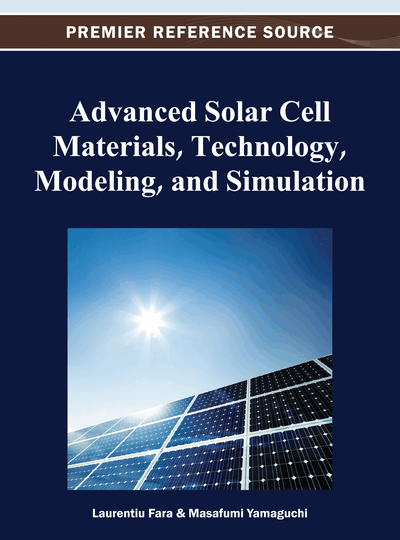# Intermediate Band Solar Cells: Modeling and Simulation

DOI: 10.4018/978-1-4666-1927-2.ch011

## Abstract

The Intermediate Band Solar Cell (IBSC) is a novel photovoltaic device with the potential of surpassing the efficiency limit of conventional solar cells. It is based on a new class of materials characterized by the insertion of a collection of energy levels within the material bandgap. These levels act as the so-called Intermediate Band (IB) and cause a larger portion of the solar spectrum to be useful for photovoltaic conversion. Sub-bandgap photons can ideally be collected via two-step absorption mechanisms through the IB and thus enhance the photogenerated current without a significant voltage degradation. In this chapter, the authors show the state of the art of the modeling and simulation within the IBSC research field.
Chapter Preview
Top

## 1. Introduction

The solar energy utilization is inherently and fundamentally inefficient because it is very diluted compared to other sources of energy. This causes the exploitation of the Sun as a direct source of energy for cheap electricity production to be very challenging.

There are two ways of reducing the cost of Photovoltaics (PV): 1) reducing the solar cell manufacturing cost and/or 2) boosting the solar cell efficiency, which implies PV concepts with a high efficiency ceiling. Both strategies cannot be compared under the same standards, since the cost associated to the PV module is expected to be approximately half of the price of the whole PV power plant (the Balance-of-System accounting for the other half) and the solar cell cost (in current flat panels) approximately accounts, in average, for the 60% of the PV module cost (del Cañizo, del Coso, & Sinke, 2009). This implies that any PV technology cost reduction only affects approximately 30% of the overall cost; while the efficiency enhancement strategy affects the entire cost of the investment. Furthermore, the marginal cost of operation and maintenance can be neglected in PV and therefore, the generation cost of solar electricity ultimately depends on the electricity produced and thus, on the efficiency.

One of the paths leading to a possible PV breakthrough is the Intermediate Band Solar Cell (IBSC) concept, for which a limiting efficiency as high as 63.2% (Luque & Martí, 1997) has been calculated, to be compared to the 40.7% of a conventional solar cell (Araujo & Martí, 1994; Shockley & Queisser, 1961). The IBSC is based on the so-called Intermediate Band (IB) materials, which can be regarded as a new type of materials engineered so that an energy band or a collection of energy levels are inserted within the semiconductor bandgap. These materials are capable of absorbing sub-bandgap photons, which otherwise would be useless for PV conversion. These sub-bandgap photons are collected via two-step electronic transitions through the IB, thus enabling the pumping of an extra electron flux from the Valence Band (VB) to the Conduction Band (CB). This mechanism is sketched in Figure 1. The first step, or sub-bandgap transition, gIV, represents the pumping from the VB to the IB, through the bandgap EH. The second step is denoted as gCI and represents the electron pumping from the IB to the CB (through the bandgap defined as EL). The conventional transition from the VB to the CB is denoted as gCV in the figure (and occurs through EG). The choice of the location of the IB closer to the CB and therefore in the upper half of the host material bandgap has been arbitrarily made in this example. In this respect, the IB concept is symmetric from a theoretical point of view (EL can be above EH and vice versa). In many practical cases however (particularly in the implementation with QDs), as it will be seen later, the IB will be located in the upper half.

Figure 1.

Simplified band diagram of an IBSC where the QFLs associated to each of the bands (VB, IB, and CB) are represented together with their corresponding transitions and bandgap distribution

The overall effect of this double absorption causes a larger portion of the solar spectrum to become useful for the extraction of carriers. At the same time, these photogenerated carriers must preserve their electrochemical potential. As a result, the IBSC has the potential to achieve a short circuit current (ISC) enhancement without a significant degradation of the open circuit voltage (VOC). The latter occurs under the assumption that three electron gases coexist, each of them associated to each of the three bands: VB, IB and CB. Out of the equilibrium, these electron gases are defined by their own Quasi-Fermi Level (QFL) and they are respectively denoted as εFh, εFIB and εFe, in Figure 1. The existence of three well defined and separated electronic populations associated to each band is on the basis of a VOC not limited by any of the sub-bandgaps (EL or EH, in this case), but only limited by the host or barrier material bandgap, EG.

## Complete Chapter List

Search this Book:
Reset